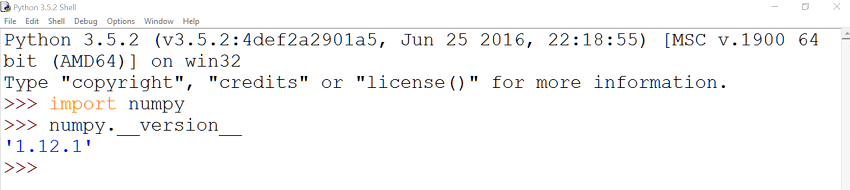# Introducing NumPyNumeric is a package that was originally developed by Jim Hugunin. It is considered the ancestor of NumPy, a Python library and an open-source project created by Travis Oliphant which stands for Numerical Python. Travis created NumPy by incorporating features of the Numarray package into Numeric.

The fundamental idea of NumPy is support for multidimensional arrays. So NumPy can be considered as the base for numerical computing in Python, and has been created to enable Python to be used in solving mathematical and scientific problems. The NumPy module provides us with hundreds of useful mathematical functions in addition to constants such as the base of natural logarithms (`e`) and pi (`π`).

This tutorial shows how we can use NumPy to work with multidimensional arrays, and describes the ndarray object, a fundamental object of the library.

## Installing NumPy

Since Python doesn’t come bundled with NumPy, the first step to use this library is to go ahead and install it. This can be simply done by running the following command in your command prompt:

To make sure that NumPy was installed successfully, run the following commands in Python’s IDLE:

If the `import` statement at least runs successfully, then you are all set!

## The ndarry Object

The `ndarray` is a fundamental object of NumPy. This object is an N-dimensional array, meaning that it contains a collection of elements of the same type indexed using N (dimensions of the array) integers.

The main attributes of `ndarray` are data type (`dtype`), `shape`, `size`, `itemsize`, `data`, and `ndim`. Let’s learn what each attribute means through an example.

In this example we are going to use NumPy to create an array. I will not give the dimensions of the array and other information, as we will see that using the above attributes.

Notice that we used the `array` function to create an array. The output of the above script is as follows:

### dtype

The `dtype` attribute can be run as shown in the following statement:

The above statement will return `int32` as the data type. This means that the elements of the array are of type `int32`. I’m getting `32` as I’m using a 32-bit Python. If you are using a 64-bit Python, you will get `int64`, but we are dealing with integers at the end.

Since NumPy is used in scientific computing, it has many data types, as shown in the documentation. Notice that the majority of the NumPy data types end with a number, which indicates the number of bits associated with that type (this was mentioned briefly in the above paragraph).

The following examples show how we can convert from one type to another:

The above statements return the following:

Although we can convert from one type to another, it is important to note that we cannot convert a complex number into an integer or a float.

### shape

The shape attribute returns a tuple of the array dimensions. So the following statement:

will return `(4,4)`, meaning that our array is composed of `4` rows and `4` columns.

### size

The `size` attribute returns the number of elements in the array. Thus, if we type:

we will get `16` as the result, meaning that we have `16` elements in our array.

### itemsize

The `itemsize` attribute returns the size of one array element in bytes. The following statement:

will return `4`. This means that each array element is of size 4-bytes.

### data

The `data` attribute is a Python buffer object that points to the start of the array’s data. If we type the following:

we will get the following: .

### ndim

The attribute `ndim` will return the number of the array dimensions. So typing the following statement:

will return `2`, that is the array consists of two dimensions.

After understanding what the different `ndarray` attributes mean, let’s take a look at some more examples of using `ndarray`.

#### Example 1

Say we want to create a new array with one row and five columns. We would do that as follows:

The output of the above statement is: `[1 2 3 4 5]`.

#### Example 2

In this example, I’m going to rewrite the first example in this tutorial, but using `[ ]` instead of `( )`, as follows:

#### Example 3

This example shows how we use a structured data type, where we declare the field name and the corresponding data type:

If we `print(data_type)`, we will get the following:

Scroll to Top